Next: Differentiation Circuit Up: Mathematical Operations Previous: Mathematical Operations

### Current Summing Amplifier

Consider the current-to-voltage converter shown in figure 6.7. Applying our ideal amplifier rules gives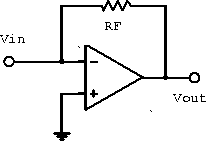Figure 6.7:  Current-to-voltage converter.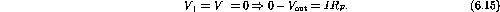Therefore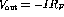and the circuit acts as a current-to-voltage converter.

Figure 6.8 shows several current sources driving the negative input of an inverting amplifier. Summing the current into the node gives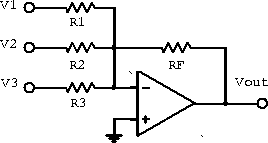Figure 6.8:  Current summing amplifier.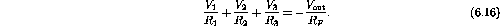Therefore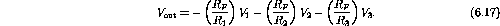If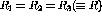, we have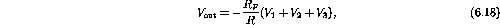and the output voltage is proportional to the sum of the input voltages.

For only one input and a constant reference voltage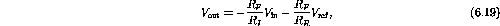where the second term represents an offset voltage. This provides a convenient method for obtaining an output signal with any required voltage offset.

Doug Gingrich
Tue Jul 13 16:55:15 EDT 1999